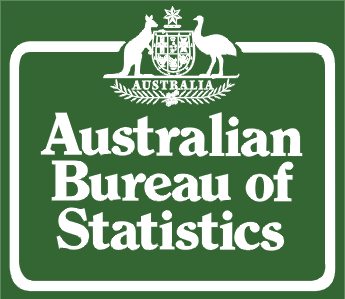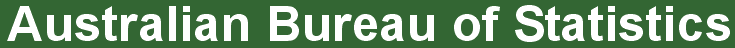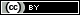ABS Home
 Statistical Language - What are Variables?What are Variables?

This animation explains the concept of variables. If you are unable to access the video a Transcript (.doc 30kb)has been provided. The animation requires Adobe Flash Player to run. The animation contains no audio.

What is a variable?

 A variable is any characteristics, number, or quantity that can be measured or counted. A variable may also be called a data item. Age, sex, business income and expenses, country of birth, capital expenditure, class grades, eye colour and vehicle type are examples of variables. It is called a variable because the value may vary between data units in a population, and may change in value over time. For example; 'income' is a variable that can vary between data units in a population (i.e. the people or businesses being studied may not have the same incomes) and can also vary over time for each data unit (i.e. income can go up or down). What are the types of variables? There are different ways variables can be described according to the ways they can be studied, measured, and presented. Numeric variables have values that describe a measurable quantity as a number, like 'how many' or 'how much'. Therefore numeric variables are quantitative variables. Numeric variables may be further described as either continuous or discrete: A continuous variable is a numeric variable. Observations can take any value between a certain set of real numbers. The value given to an observation for a continuous variable can include values as small as the instrument of measurement allows. Examples of continuous variables include height, time, age, and temperature. A discrete variable is a numeric variable. Observations can take a value based on a count from a set of distinct whole values. A discrete variable cannot take the value of a fraction between one value and the next closest value. Examples of discrete variables include the number of registered cars, number of business locations, and number of children in a family, all of of which measured as whole units (i.e. 1, 2, 3 cars). The data collected for a numeric variable are quantitative data. Categorical variables have values that describe a 'quality' or 'characteristic' of a data unit, like 'what type' or 'which category'. Categorical variables fall into mutually exclusive (in one category or in another) and exhaustive (include all possible options) categories. Therefore, categorical variables are qualitative variables and tend to be represented by a non-numeric value. Categorical variables may be further described as ordinal or nominal: An ordinal variable is a categorical variable. Observations can take a value that can be logically ordered or ranked. The categories associated with ordinal variables can be ranked higher or lower than another, but do not necessarily establish a numeric difference between each category. Examples of ordinal categorical variables include academic grades (i.e. A, B, C), clothing size (i.e. small, medium, large, extra large) and attitudes (i.e. strongly agree, agree, disagree, strongly disagree). A nominal variable is a categorical variable. Observations can take a value that is not able to be organised in a logical sequence. Examples of nominal categorical variables include sex, business type, eye colour, religion and brand. The data collected for a categorical variable are qualitative data. Types of variables flowchart:Privacy | Disclaimer | Feedback || © Copyright| Sitemap| Online Security